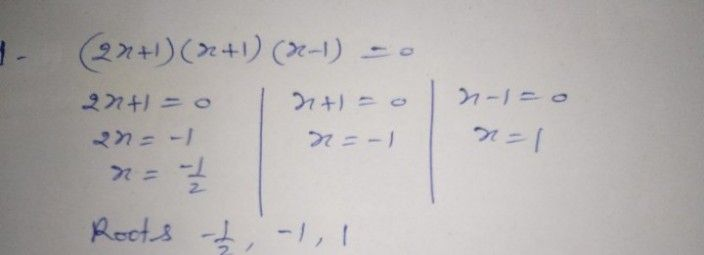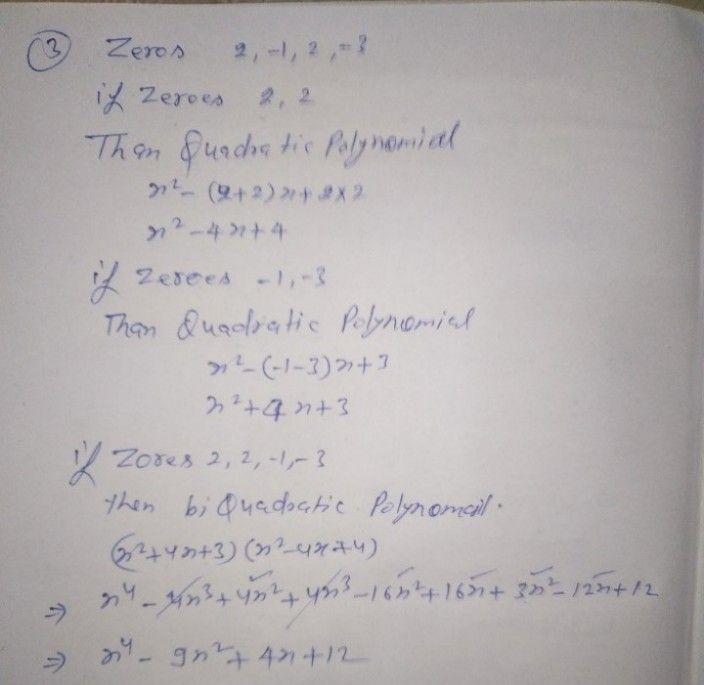Symbol
ProblemLearning Task 3. A. Perform as instructed $1.I$ Find the roots of $\left(2x+1\right)\left(x+1\right)\left(x-1\right)=0$ - $2.$ Find the zeros of the polynomial equation $x^{4+2x3-13x2-14x+24}=0$ $y2,1and-2$ 3. Find the polynomial equation whose zeros are 2 of multiplicity 3. Find the equation if the $2c03$ are $2,-1,2,-3$ B. Answer the following questions. $1.$ What do you observe about the relationship between the number of roots and the degree of a polynomial? 2. What generalization can you make when polynomial equation $1s$ expressed $29$ a product of linear factors? $3.1$ How does the "Rational Root corem" and "Factor Theorem" helps you in solving polynomial equation?
10th-13th grade
Other
Search count: 116
SolutionQanda teacher - RASHMIKAwe are only one question allow at time by qanda policy
if you have more questions so pls ask on preferred match on my profile
thanks
best of luckStudent
thanks